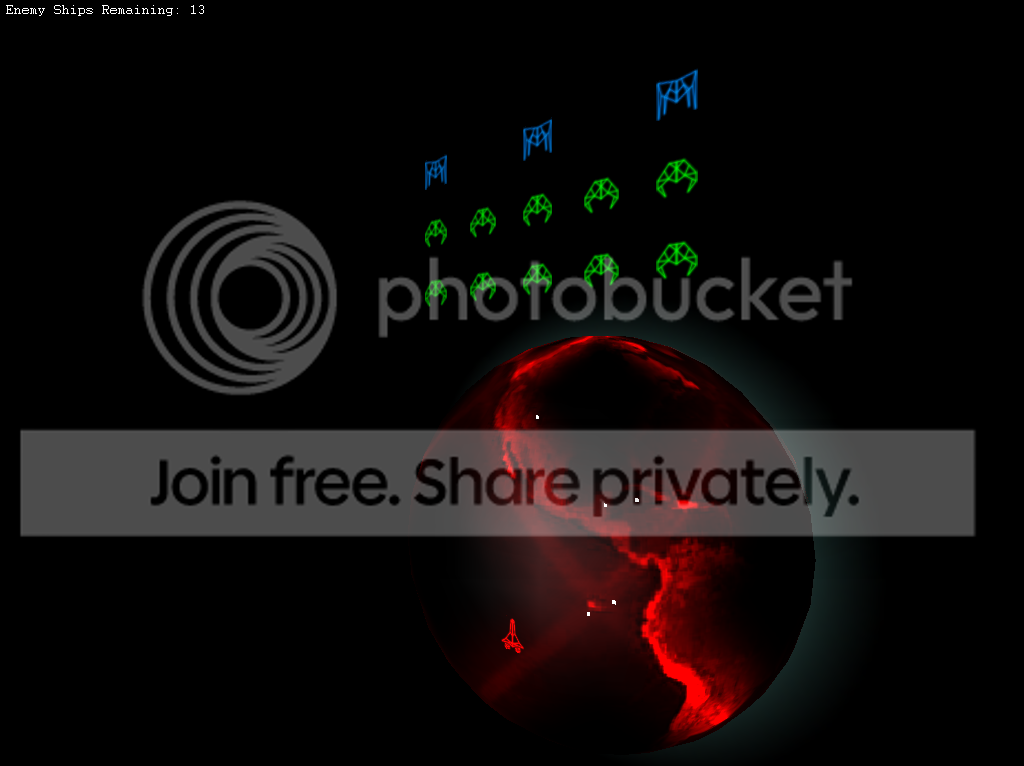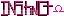-=+=- -=+=- -=+=- -=+=- -=+=- -=+=- -=+=- -=+=- -=+=- -=+=- -=+=- -=+=- -=+=- -=+=- -=+=- -=+=- -=+=- -=+=- -=+=- -=+=- -=+=- -=+=- -=+=- -=+=- -=+=- -=+=- -=+=- -=+=- -=+=- -=+=- (c) WidthPadding Industries 1987 0|302|0 -=+=- -=+=- -=+=- -=+=- -=+=- -=+=- -=+=- -=+=- -=+=- -=+=- -=+=- -=+=- -=+=- -=+=- -=+=- -=+=- -=+=- -=+=- -=+=- -=+=- -=+=- -=+=- -=+=- -=+=- -=+=- -=+=- -=+=- -=+=- -=+=- -=+=-
SoCoder -> Showcase Home -> Remakes

SchererererCreated : 23 May 2008

System : Windows
Language : Blitz

I:CN v1.0.0.0
ScreenshotsDefend the red planet against the neon forces of evil!

Current Features:
• 4 Intense Levels
• Pretty Effects

Friday, 23 May 2008, 17:07
HoboBenNeat! Wasn't there supposed to be music though (according to the readme?)
Friday, 23 May 2008, 21:19
SchererererWell, yes, except the music made it be 12 meg!... Sooo i took it out!Tuesday, 27 May 2008, 12:43
blanko1324Really hard to play...but it's cool to watch.